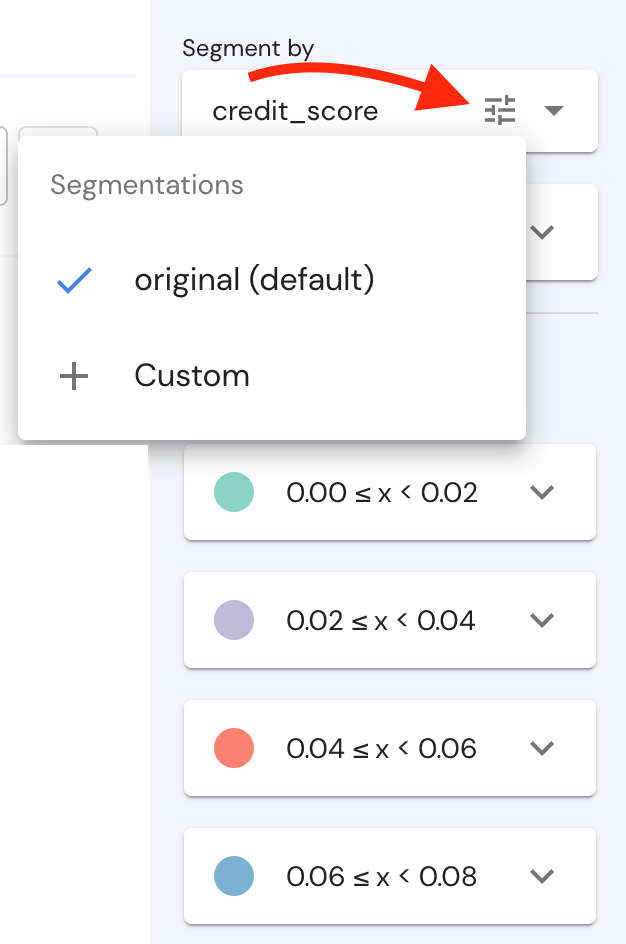# Numeric Fields Segmentation

A key feature in Mona is its ability to deeply segment the data to find specific anomalies and create reports in subpopulations of your data.

While segmenting the data according to values of string or boolean fields is trivial, numeric field values can be bucketed in infinite ways. Using “segmentation” objects within numeric field configurations, Mona can be configured to segment the numeric field’s values in several ways, as described on the possible parameters below.

No matter what type of segmentation config you use, an automatic "MISSING" segment will always be created, consisting of all the contexts which have no value for the given field.

The key of a Segmentation object is its name, can contain the following keys:

• default: Dictates whether this is the default segmentation for the specific field. Please note:
• Only one segmentation is allowed to have "default"=true
• This key is a must in the sense that one segmentation (exactly) should have this key equal to true. On all other segmentations, it is not necessary to add this key with the false value.
• discrete: This key is used in order to segment numeric data in a discrete manner, i.e. each numeric value will be assigned into a separate bucket.
• min_value: The lower bound for segmentation (inclusive). If no value is set, Mona will not define a lower bound for this field.
• max_value: The upper bound for segmentation (exclusive).
• should_log: A boolean designation telling Mona to segment on a logarithmic scale with base 2. Mona will create logarithmic buckets for positive values above 1 and negative values below -1. For example, the value 7 will be bucketed in the 4<=x<8 bucket. Values between -1 to 1 will be segmented into the buckets -1<=x<0 and 0<=x<1. For example, the bucket of 0.6 will be 0<=x<1. This field cannot be used together with number_of_buckets, bucket_size, or bucket_boundaries.
• number_of_buckets: A numeric value telling Mona to segment to fixed-sized buckets on a linear scale between min_value to max_value. Mona will create segments roughly in the size of (max_value-min_value)/number_of_buckets. For example, if min_value = 0, and max_value = 9, and number_of_buckets = 3, then the segments will be 0<=x<3, 3<=x<6, 6<=x<9. This field requires min_value and max_value.
• bucket_size: A numeric value telling Mona to segment on a linear scale to buckets of this size. Mona will create segments in the size of bucket_size starting at bucket_offset. For example, if bucket_size = 4, then the segments will be 0<=x<4, 4<=x<8, and so on.
• bucket_offset: (default=0) The offset to start bucketing when using bucket_size.
• bucket_boundaries: An array of numeric values telling Mona to segment on buckets between each two consecutive values. For example, if bucket_boundaries = [-1, 3, 10, 20, 25], then the segments will be -1<=x<3, 3<=x<10, and so on.

A short example using a field called "sentiment_score" may make this easier to grasp:

``````{
"sentiment_score": {
"source": "out.sentiment_score",
"type": "numeric",
"segmentations": {
"log_buckets_zero_to_15k": {
"min_value": 0,
"max_value": 15000,
"should_log": true
},
"linear_buckets_zero_to_15k": {
"default": true,
"min_value": 0,
"max_value": 15000
},
"discrete_buckets_no_boundaries": {
"discrete": true
}
}
}
}
``````

Note how we allowed three different kinds of segmentation while directing Mona to segment this field using "linear_buckets_zero_to_15k" by default.

### Dynamic Segmentation

The segmentation sidebar on the investigations page allows users to change the segmentation of a numeric field without changing via the config. A click on the "dynamic segmentation" button in the segment_by tab, a menu will appear where users can choose to create a new custom segmentation.Once created, this segmentation can either be set as saved preset segmentation, the default segmentation or not saved and relevant only until the page is refreshed.